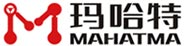#+86 138-2720-6784 CN

# Summary of calculation methods for sheet metal bending and unfolding

Publication time：2022/03/29 08:56:55 Reading volume：315 Source： Jiangxi MAHATMA Intelligent Technology Co., Ltd

90 degree bending: the simplest calculation method of bending coefficient belongs to the empirical formula of 90 degree bending coefficient: calculation method of 1.7 times material thickness. Used in 90 sheet metal bending, one right angle bend minus 1.7 times the material thickness. For example, if the material is 1mm iron plate, the bending angle is 90 degrees, and the bending dimensions are 100mm and 50mm respectively, the calculation and expansion method is: 100 + 50-1.7 = 148.3mm. The calculation is the expansion length. This 1.7 is said to be 1.6 or 1.65 times. Yes, it can be slightly adjusted. Because the bending dies used by each sheet metal factory are not exactly the same, there are slight errors, so they can be used without adjustment, and they can also be adjusted slightly if they have high requirements.

Non 90 degree bending: sheet metal bending is not only 90 degree bending, but also non 90 degree bending. Is there the simplest calculation method?

This is not true. There is a calculation formula for accurately calculating the non-90 degree bending coefficient, which is to use the concept of neutral layer to calculate the arc length of the arc during bending, and finally calculate the bending coefficient. For a special angle, the bending coefficient can be calculated by a simple method. When the sheet metal bending angle is 135 degrees, the bending factor can be reduced by 0.5 times the material thickness. For example, if the material is 1mm iron plate, the bending angle is 135 degrees, and the bending dimensions are 100mm and 50mm respectively, the calculation expansion method is: 100 + 50-0.5 = 149.5mm. Other sheet metal thickness can also be calculated by this method. Only applicable to 135 degrees, other angles are not available.

Sheet metal hem: there is also a special angle bending in sheet metal bending, that is, sheet metal hem, also known as dead edge, which can be calculated by a simple method. The bending factor is equal to 0.4 times the sheet metal thickness. For example, if the material is 1mm iron plate, the bending is the dead edge, and the bending dimensions are 100 and 10 respectively, the calculation and expansion method is: 100 + 10-0.4 = 109.6mm.

### 联系我们 更多 +Product 135-2852-8896 Telephone